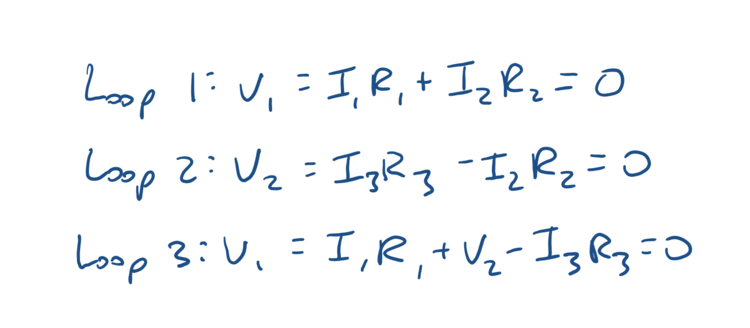# PHYS 1120 / 2020 Midterm 2 Study Guide

## Voltage

Voltage from a point particle:Voltage from an electric field:* The only distance Δd that matters is the distance that is parallel to the electric field *

How voltage is related to work/energy:If you need to find a particle’s speed from its voltage or energy, use the work-energy theorem from Physics 1:## Capacities

For a parallel-plate capacitor, THE ONLY THING THAT CHANGES CAPACITANCE IS A CHANGE IN THE AREA OF THE PLATES OR THE DISTANCE BETWEEN THE PLATES!!!A capacitor is related to the charge on its plates and the voltage between its plates by* Q and V can change each other but they can’t change C!!! *

## Resistors + Simple Circuits

Resistance of a resistor is determined by its length and cross-sectional area:In series voltage adds and current is constant!In parallel voltage is constant and current adds!## Brightness of Bulbs

Highest brightness means most powerChoose the equation with the most stuff that’s constant (i.e. brightness of bulbs in parallel are P=V^2/R since voltage is constant in parallel)

Real quick going back to energy in capacitors cuz I forgot:Again, use the equation that has the most stuff that’s constant: connected to a battery => constant voltage, not connected to a battery => constant Q

## Kirchoff’s Law

The node law: At a 3-way intersection in a circuit, current entering the intersection equals current leaving the intersection!so here:The loop law: If you trace any loop around a circuit the total voltage has to equal zero!

Example:+ It doesn’t matter the direction of your loop, but you have to follow these rules:

1. Voltage going in same direction of loop is positive

2. Voltage going opposite of the loop is negative

3. Current going with the loop over a resistor is negative

4. Current going opposite to the loop over a resistor is positive## Alex Koek

January 11, 2020

View Profile

#### Get in Touch

Thanks! We'll reach out shortly.